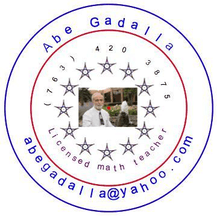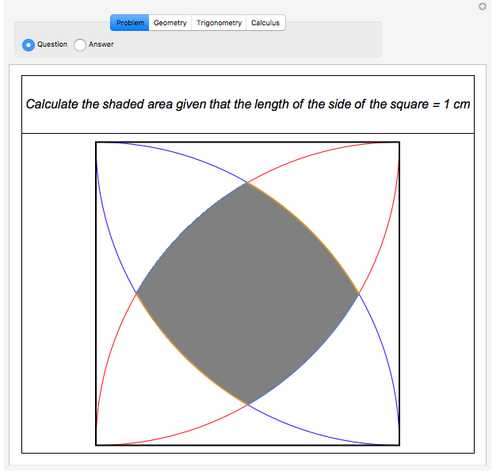#Wolfram Summer School

# AlumniEducational Computational X

Class of 2019

## Bio

Abe graduated as an Electrical Engineer from Cairo University, Cairo, Egypt, in 1966. After working for several years in Cairo, he went to Germany and worked and studied there. In 1979, Abe earned a Master’s Degree in Aerospace Engineering (Dipl. Ing.) from Bremen University. While working full time at Control Date in Minnesota, Abe earned a Master’s Degree in International Management (MIM) from the University of St. Thomas in 1989. In 1996, Abe joined the University of Minnesota to obtain a teaching license in Mathematics K–12, which is valid until 2020. Since 1990, while Abe was on a Master’s Degree program at the University of Minnesota, Abe was involved with Mathematica (Version 2.5 at that time). Abe used the Wolfram Language in the classroom in order to clarify and to visualize the mathematical concepts and to build students’ confidence. You can see some of the programs that Abe wrote at demonstrations.wolfram.com. Abe presented “Effective Teaching with Mathematica in Algebra, Geometry, and in Calculus” at teachers’ conferences in Duluth, Minnesota; Green Lake, Wisconsin; and Honolulu, Hawaii. Abe strongly believe that the Wolfram Language is the most effective tool for teaching and learning. It saves teachers’ time as well as students’ time while engaging all students and helping them understand mathematical concepts in depth. The Wolfram Language should be the main tool in all schools all over the countries for real teaching and learning among other real life activities.

## Project: A Collection of Educational Demonstrations### Goal

Create challenging mathematical projects for high-school students. The main goal is to clarify and illustrate the mathematical concepts in real-life applications. Using Manipulate, one can display the questions as well as clarify the answers step by step. By visualizing the mathematical concepts, students will have in-depth understanding of the concepts.

### Summary of Results

I learned how to create a general case for a particular math problem and submitted a Demonstration on a problem calculating a shaded area among four curves in a square that displays the solution in three different categories: the seven steps for a geometric solution plus a summary, two trigonometric solutions and one solution using integration.

### Future Work

Create more mathematical challenges using the Wolfram Language.

APPLY NOW